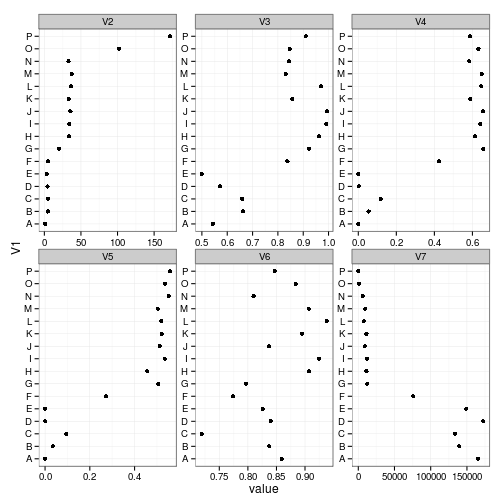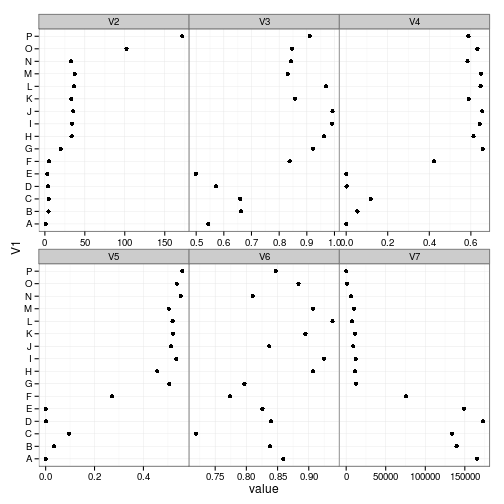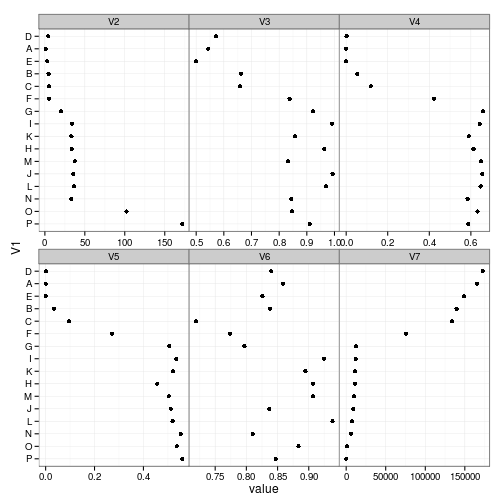## “Squeezing” ggplot plots created with facet.wrap

Version 2013-01-08 10:10:47

I'm often in a situation where I want to create a bunch of subplots/facets with identical $$y$$ axes but differently scaled $$x$$ axes. I can do this with facet_wrap, but then I feel the need to get rid of the superfluous sets of $$y$$-axes on the internal facets, and squash the vertical interpanel spaces (this can't be done with theme(panel.margin), which applies equally to all margins).

Get packages:

library(ggplot2)
theme_set(theme_bw())
library(reshape2)
library(grid)


These data are the anonymized/jittered version of some data I received in another context recently …

dat <- read.csv("squeezeAxisdat.csv")
mdat <- melt(dat,id.var=1)


Using https://groups.google.com/forum/?fromgroups=#!topic/ggplot2/cnVDSyobPy4 as a starting point, I came up with this function:

source("squashGrid.R",keep.source=TRUE)
squashGrid

## function(g) {
##     g2 <- ggplotGrob(g)
##     Lnames <- g2$layout$name
##     pstr <- "^panel-[0-9]+"
##     totpanel <- max(as.numeric(gsub("panel-","",grep(pstr,
##                             Lnames,value=TRUE))))
##     panels <- g2$layout[grepl(pstr,Lnames),] ## nrow <- length(unique(panels$t))
##     ncol <- length(unique(panels$l)) ## ## find interior facets (all but leftmost column) ## squash_facets <- matrix(seq(nrow*ncol),nrow=nrow,byrow=TRUE)[,-1] ## squash_facets <- squash_facets[squash_facets<=totpanel] ## ## find columns corresponding to axis labels and vertical margins for all but leftmost column ## ## preserve first three columns (L margin, margin and tick labels for first column); ## ## preserve plot columns ## totcol <- length(g2$width)
##     squash_cols <- sort(outer(0:1,seq(5,length.out=ncol-1,by=3),"+"))
##     ## find position of left axes of facets
##     left_axis_idx <- grep("axis_l-",Lnames)
##     axis_nums <- as.numeric(gsub("axis_l-","",Lnames[left_axis_idx]))
##     left_axis_idx <- left_axis_idx[axis_nums %in% squash_facets]
##     ## overwrite them with "null" graphical objects
##     g2$grobs[left_axis_idx] <- replicate(length(left_axis_idx),nullGrob(),simplify=FALSE) ## ## squash spacing of columns corresponding to panel margins and axis labels ## g2$widths[squash_cols] <- replicate(length(squash_cols),
##                                         unit(0,"cm"),simplify=FALSE)
##     grid.draw(g2)
## }


This function automatically finds the interior (all-but-leftmost-column) facets and the columns corresponding to the spaces between them and eliminates them.

The original plot:

(g1 <- ggplot(mdat,aes(x=value,y=V1))+geom_point()+
facet_wrap(~variable,scale="free"))The squashed version:

squashGrid(g1)It may be easier to read if we order by increasing V7:

mdat2 <- melt(dat2 <- transform(dat,V1=reorder(V1,V7)),id.var=1)
squashGrid(g1 %+% mdat2)## To do

• Is there a better name for this?
• Could fairly easily extend this to allow for bottom label/space squashing, or for only removing tick marks and not removing spaces?